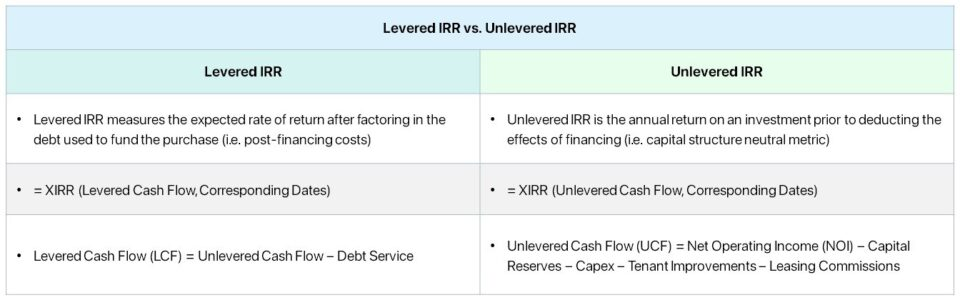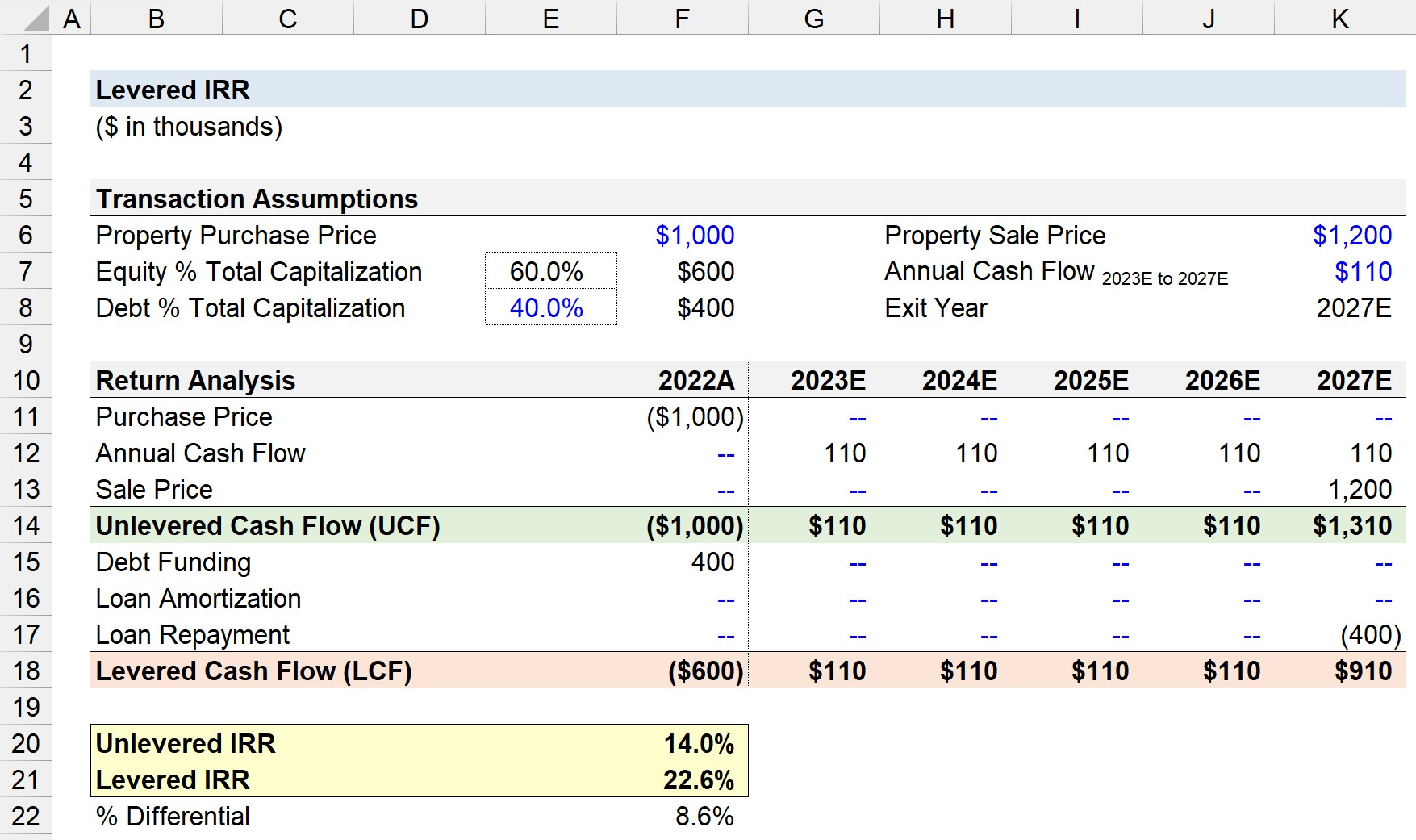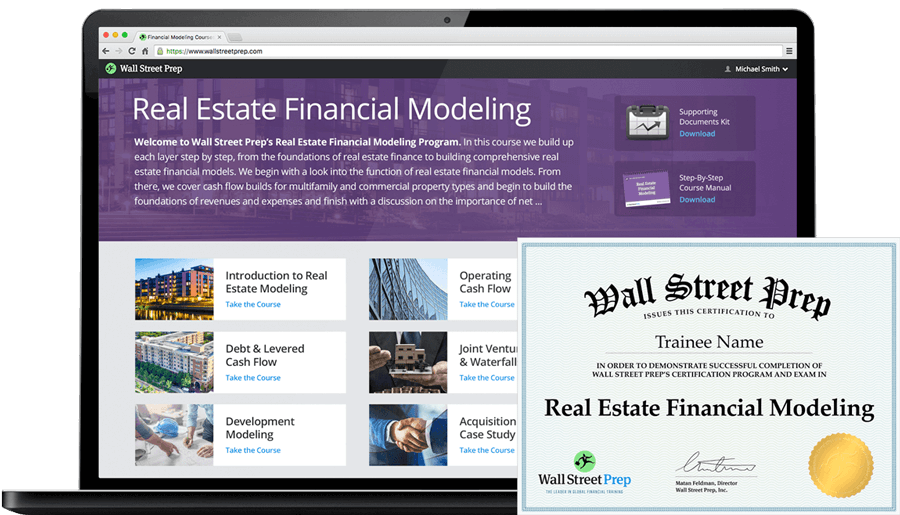Welcome to Wall Street Prep! Use code at checkout for 15% off.# Levered IRR

Guide to Understanding Levered IRR## How to Calculate Levered IRR?

The levered IRR, or “equity IRR”, is a method to determine the potential yield earned on a real estate investment, whereby the return factors in the effects of leverage.

In financing, the term “leverage” refers to the reliance on debt to fund the purchase price of an investment – i.e. the act of borrowing funds from lenders such as banks or institutional investors – to reduce the required equity contribution.

The cash flow generated by the investment property remains relatively identical regardless of the financing structure, yet the equity contribution from the investor is lower if debt is used to finance the transaction.

Hence, the levered IRR of an investment property exceeds the unlevered IRR in practically all cases.

The greater the spread between the levered IRR and unlevered IRR, the more reliant the anticipated investment returns are on leverage.

The drawback to leverage is the potential downside risk created by placing a debt burden on the property.

In effect, the credit risk of the investment property rises – in particular, a greater proportion of debt coincides with a higher risk of default, such as missing a required mortgage payment or periodic interest obligation.

## How Leverage Impacts Returns in Real Estate Investing?

The real estate market, especially for commercial properties, is a highly levered asset class, where it is common for the funding structure of real estate projects to consist of debt financing.

Most investments in the commercial real estate (CRE) market are financed partially by debt capital because the purchase prices are set higher, and the potential to earn an outsized return is much greater if leverage is used.

The less equity required to be contributed by the real estate investor, the higher the internal rate of return (IRR) – all else being equal.

The investment returns in the commercial real estate (CRE) market stem primarily from two sources:

• Cash Flow Growth → The growth in cash flow (or income) generated by a property stems from improvements in operating efficiency, in which more value is extracted from tenants (e.g. increase in rent prices). Further, there should be an expansion in the margin profile of the investment property, such as its NOI margin, from these initiatives to reduce costs.
• Capital Appreciation → The capital appreciation concept refers to the value of an investment property increasing relative to the original purchase cost. Similar to achieving multiple expansion in LBOs, where the exit multiple is higher than the purchase multiple, the sale price at exit exceeds the acquisition price on the date of initial purchase.## Levered IRR Formula

The internal rate of return (IRR) measures the percent yield received on an investment on an annualized basis.

The formula to calculate the levered IRR in Excel is as follows.

=XIRR(Levered Cash Flow, Corresponding Dates)

The internal rate of return (IRR) is determined using the “XIRR” function in Excel, which comprises two arrays:

• Cash Flow → The cash flow array is the net differential between the cash “inflows” and cash “outflows” in each period. However, the distinction between the unlevered and levered IRR is that the latter factors in the debt funding, so the cash flow used here is the levered cash flow.
• Corresponding Dates → The second array is the specific dates that coincide with each net cash flow, i.e. to match the timing.

While the mechanics of computing IRR in Excel are practically the same for the levered and unlevered IRR, understanding the distinction between unlevered cash flow and levered cash flow is critical.

## Unlevered Cash Flow vs. Levered Cash Flow Formula

The unlevered cash flow of a real estate property uses the remaining cash before deducting debt payments, such as interest or mortgage payments.

The unlevered cash flow is the net cash flow of a property before financing items (i.e. capital structure neutral).

Since the unlevered cash flow reflects only the operating activities of the property, the metric facilitates comparisons between the operating performance of comparable investment properties, in which only the core operating activities are considered.

Unlevered Cash Flow (UCF) = Net Operating Income (NOI)  Capital Reserves  Capex  Tenant Improvements  Leasing Commissions

Where:

• Net Operating Income (NOI) → NOI measures a property’s operating cash flow prior to deducting debt service (i.e. capital structure neutral metric) and capital costs.
• Capital Reserves → Capital reserves are funds that are set aside to meet future capital expenditures (Capex) requirements and capital improvements
• Capex → Capital expenditure or “Capex” refers to long-term spending on property acquisitions, property upgrades, and property repairs.
• Tenant Improvements (TI) → Tenant improvements refer to upgrades made to a property by a landlord to accommodate the specific needs or requests of a tenant.
• Leasing Commissions → Leasing commissions are the fees paid to real estate agents or brokers as compensation for successfully introducing tenants to a given rental property (post-completion of lease).

Unlike unlevered cash flow, the levered cash flow metric reflects the net cash flow of a property post-financing.

To shift from the unlevered cash flow to the levered cash flow metric, the subsequent adjustment consists of deducting debt services, such as the annual mortgage payment and interest.

Levered Cash Flow (LCF) = Unlevered Cash Flow  Debt Service

Where:

• Unlevered Cash FlowNet Operating Income (NOI) – Capital Reserves – Capex – Tenant Improvements – Leasing Commissions
• Debt Service → Annual Mortgage Payments + Interest Expenses

Once the debt service is subtracted from unlevered cash flow to arrive at the levered cash flow of a property, we are left with the post-financing, residual net cash flow attributable to only equity stakeholders.

## Levered IRR vs. Unlevered IRR: What is the Difference?

1. Levered IRR → The levered IRR is the expected rate of return after factoring in the leverage used to fund the investment. Why? The required equity contribution by the real estate investor is reduced because of the financing, yet the cash flow remains the same – causing the yield earned by the investor to rise, all else being equal. The initial outflow of cash is the equity contribution of the investor (i.e. Purchase Price – Initial Debt). The stream of cash flows measured to calculate the levered IRR is the net operating income (NOI) of the property minus the annual debt service, such as the interest payment owed on an outstanding loan.
2. Unlevered IRR → On the other hand, the unlevered IRR measures the return on an investment but neglects the effects of financing. The initial outlay of cash is the total purchase price of the property since the implicit assumption in the unlevered IRR metric is that the purchase was paid for entirely using cash (i.e. all-equity). The projected cash flow metric measured in the unlevered IRR metric is NOI, an unlevered capital structure neutral metric. In short, the unlevered IRR is the expected rate of return, assuming no non-equity financing was used to fund the investment. The unlevered IRR only considers the cash flows produced by the property investment itself.

Therefore, the levered IRR is the rate of return after considering the leverage used to finance the original purchase, whereas the unlevered IRR represents the return on an investment without debt financing (i.e. independent of the capitalization).

If sufficiently meeting the minimum target return is contingent on the substantial use of leverage, the investment opportunity may be considered as riskier and might not be worth pursuing.

Simply put, using debt as a primary strategy to create value by itself is far riskier relative to plans to facilitate growth in net operating income (NOI) and capital appreciation from identifying favorable market trends or implementing improvements to properties.

## Levered IRR Calculator

We’ll now move to a modeling exercise, which you can access by filling out the form below.#### Excel TemplateSubmitting...

## Levered IRR Calculation Example

Suppose a real estate investor acquired a property at a purchase price of \$1 million, where the transaction was financed using 60% equity and 40% debt.

• Property Purchase Price = \$1 million
• Equity % Total Capitalization = 60.0% × \$1 million = \$600 million
• Debt % Total Capitalization = 40.0% × \$1 million = \$400 million

The closing date of the acquisition was the end of 2022 (12/31/2022). Starting in 2023, the first year in the five-year hold period, the property is projected to generate \$110k in annual cash flow from 2023 to 2027.

Further, we’ll assume the investment will be sold at the end of 2027 at a sale price of \$1.2 million.

• Annual Cash Flow = \$110k
• Exit Year = 2027E
• Property Sale Price = \$1.2 million

In the first part of our return analysis schedule, we’ll compute the unlevered cash flow (UCF) in each period.

1. The purchase price is linked to our property purchase price of \$1 million in Year 0 (“outflow”).
2. The annual cash flow for the entire forecast period is linked to the \$110k assumption cell (and anchored by pressing F4 once)
3. The sale price of \$1.2 million is linked to in the column for 2027E (“inflow”).

The sum of the three items results in the unlevered cash flow (UCF) for each year, which comes out to the following:

• Year 0, 2022A = (\$1 million)
• Years 1 to Year 4, 2023E to 2026E = \$110k
• Year 5, 2027E = \$110k + \$1.2 million = \$1.31 million

From the unlevered cash flow (UCF) section, we’ll then adjust for the three debt service items.

1. The debt funding in Year 0 will link to the \$400k used to fund the purchase (“inflow”).
2. The loan amortization will be assumed to be zero each period, i.e. no mandatory repayment of the principal until maturity.
3. The loan repayment, in which the entire principal is paid off at once, occurs in Year 5 (“outflow”).

The sum of the unlevered cash flow (UCF) per period and the three items outlined above results in the levered cash flow for our forecast.

Using the XIRR function in Excel, we can determine the unlevered IRR and levered IRR.

=XIRR(Unlevered Cash Flow, Corresponding Dates)
=XIRR(Levered Cash Flow, Corresponding Dates)

The unlevered IRR on the property investment comes out to 14.0%, while the levered IRR is 22.6%, reflecting the positive impact that leverage can have on the returns earned on an investment.

• Unlevered IRR = 14.0%
• Levered IRR = 22.6%
• % Differential = 22.6% – 14.0% = 8.6%20+ Hours of Online Video Training

### Master Real Estate Financial Modeling

This program breaks down everything you need to build and interpret real estate finance models. Used at the world's leading real estate private equity firms and academic institutions.

Inline Feedbacks